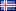ÍslenskaEnglish

23.947
4.098
1.304
1.846

# Lokaverkefni (Doktors)

Vinsamlegast notið þetta auðkenni þegar þið vitnið til verksins eða tengið í það: http://hdl.handle.net/1946/12985

Titill:
•Contributions to the meta-theory of structural operational semantics
Námsstig:
• Doktors
Höfundur:
Leiðbeinandi:
Efnisorð:
Útdráttur:
•Structural Operational Semantics (SOS) is one of the most natural ways for providing programming languages with a formal semantics. Results on the meta-theory of SOS typically (but not solely) say that if the inference rules used in writing the semantic specification of a language conform to some syntactic template then some semantic property is guaranteed to hold or some technique is applicable in order to gain some result. These syntactic templates are called rule formats.
This thesis presents four contributions on the meta-theory of SOS.
As a first contribution, (1) we offer a method for establishing the validity of equations (modulo bisimilarity). The method is developed under the vest of an equivalence relation that is suitable for mechanization, the rule-matching bisimilarity. Given a semantic specification defined in SOS and given the desired equation to check, the method runs a matching, bisimulation-like, procedure in order to determine the validity of the given equation. For the method to be applicable, the SOS specification must fit a well-known rule format called GSOS, which is fairly expressive. For instance most of the process algebras can be defined within GSOS. The method is general and, not surprisingly, might not terminate. We however show that relevant equations can be checked in finite time.
As another contribution, (2) we present rule formats ensuring that certain constants of a language act as zero elements. An example of zero element, though in the context of mathematics, is the number 0, that is a zero element for the multiplication operator _, i.e., x _ 0 = 0. Based on the design of one of the formats, we provide also a rule format for unit elements. The same number 0 is for instance an example of unit element for the sum operator +, as the algebraic law x + 0 = x is valid.
As a third contribution, (3) we offer rule formats guaranteeing the validity of the distributivity law. Examples of distributivity laws in the context of mathematics are (x + y) _ z = (x _ z) + (y _ z), i.e., the multiplication distributes over the sum, and (A [ B) \ C = (A \ C) [ (B \ C), i.e., the set intersection distributes over the union. The algebraic laws addressed by contributions (2) and (3) are considered modulo bisimilarity. In both contributions, the proposed rule formats are mostly mechanizable and some of them are also very simple to check. Nonetheless, the rule formats we offer are expressive enough to check the validity of classic zero and unit elements as well as well-known distributivity laws from the literature.
Thanks to contributions (2) and (3), now the meta-theory of SOS tackles all the basic algebraic laws, i.e., commutativity, idempotency, associativity, zero and unit elements and distributivity.
Finally, (4) we propose Nominal SOS, an SOS based framework with special syntax and primitives for the definition of languages with binders. Binders bind a name in a context in order to give it a certain meaning or to denote that a special treatment for it is required. The ordinary SOS framework lacks a dedicated account for binders. Binders, however, proliferate both in mathematics (one example is the universal quantification 8x:_) and in computer science (one example is the abstraction _x:Mof the _-calculus). We provide evidence that the framework is expressive enough to model interesting calculi. For instance, we formulated the _-calculus and the _-calculus within the framework of Nominal SOS and we established the operational correctness of these formulations with regard to the original ones. We offer a suitable notion of bisimilarity that is aware of binding and we have embarked on a study of the relationship between this notion of bisimilarity and classic equivalences from the context of the _- and _-calculus. We believe that the meta-theory of SOS is by now a mature field and times are ripe for a systematic study of the meta-theory that concerns also those phenomena that are specifically related to binders. In this respect, we believe that our framework might be a good candidate to carry out such a study.

Samþykkt:
• 12.9.2012
URI:
• http://hdl.handle.net/1946/12985

Skrár
Skráarnafn Stærð AðgangurLýsingSkráartegund
MatteoCimini_PhdThesis.pdf1.13 MBOpinnHeildartextiPDF